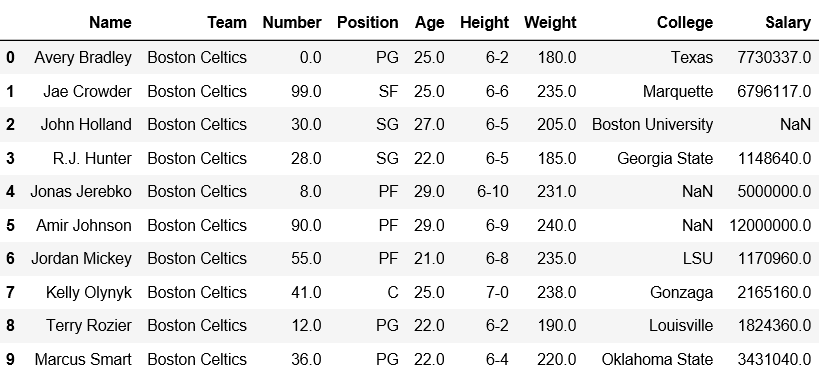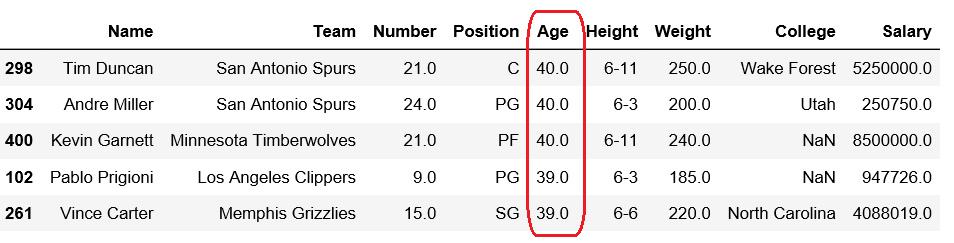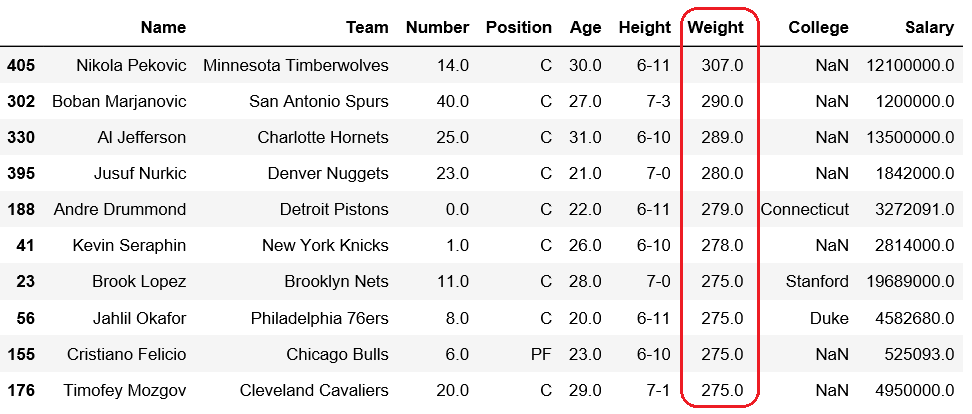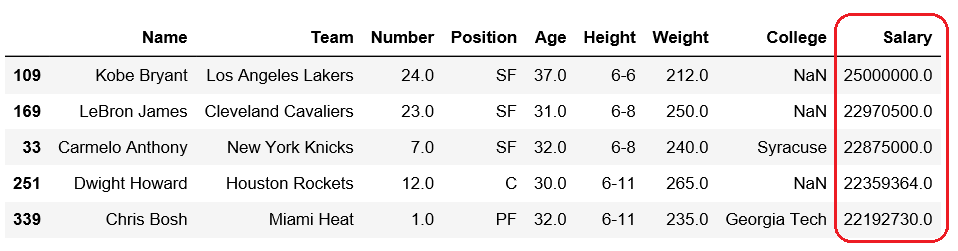# Get n-largest values from a particular column in Pandas DataFrame

Pandas DataFrame is two-dimensional size-mutable, potentially heterogeneous tabular data structure with labeled axes (rows and columns).

Let’s see how can we can get n-largest values from a particular column in Pandas DataFrame.

Observe this dataset first. We’ll use ‘Age’, ‘Weight’ and ‘Salary’ columns of this data in order to get n-largest values from a particular column in Pandas DataFrame.

 `# importing pandas module  ` `import` `pandas as pd  ` `   `  `# making data frame  ` `df ``=` `pd.read_csv(``"https://media.geeksforgeeks.org/wp-content/uploads/nba.csv"``)  ` ` `  `df.head(``10``) `Code #1: Getting 5 largest Age

 `# importing pandas module  ` `import` `pandas as pd  ` `   `  `# making data frame  ` `df ``=` `pd.read_csv(``"nba.csv"``)  ` ` `  `# five largest values in column age ` `df.nlargest(``5``, [``'Age'``]) `

Output:Code #2: Getting 10 maximum weights

 `# importing pandas module  ` `import` `pandas as pd  ` `   `  `# making data frame  ` `df ``=` `pd.read_csv(``"nba.csv"``)  ` ` `  `# Ten largest values in column Weight ` `df.nlargest(``10``, [``'Weight'``]) `

Output:Code #3: Getting 10 maximum salary

 `# importing pandas module  ` `import` `pandas as pd  ` `   `  `# making data frame  ` `df ``=` `pd.read_csv(``"nba.csv"``)  ` ` `  `# five largest values in column Salary ` `df.nlargest(``5``, [``'Salary'``]) `

Output:Whether you're preparing for your first job interview or aiming to upskill in this ever-evolving tech landscape, GeeksforGeeks Courses are your key to success. We provide top-quality content at affordable prices, all geared towards accelerating your growth in a time-bound manner. Join the millions we've already empowered, and we're here to do the same for you. Don't miss out - check it out now!

Previous
Next